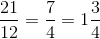# High School Math : Understanding Addition and Subtraction

## Example Questions

### Example Question #1 : Adding And Subtracting Fractions

Simplify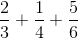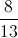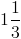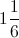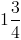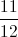Explanation:

Find the least common denominator (LCD) and convert each fraction to the LCD and then add.  Simplify as necessary.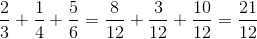The result is an improper fraction because the numerator is larger than the denominator and can be simplified and converted to a mix numeral.